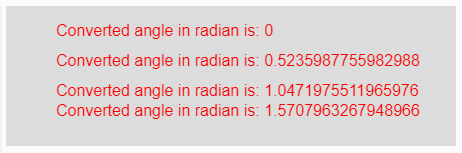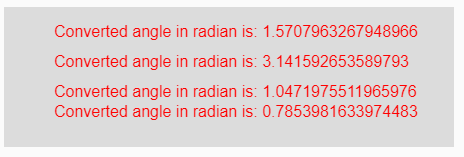The radians() function in p5.js is used to convert a given degree measurement value to its corresponding value in radians.

Syntax:

`radians( degrees )`

Parameters: This function accepts single parameter degrees which is to be converted into radians.

Return Value: It returns the converted radians value of angle.

Below programs illustrate the radians() function in p5.js:

Example 1: This example uses radians() function to convert a given degree to its corresponding radians.

 `function` `setup() {  ` `  `  `    ``// Creating Canvas size ` `    ``createCanvas(450, 140);  ` `}  ` ` `  `function` `draw() {  ` `      `  `    ``// Set the background color  ` `    ``background(220);  ` `    `  `    ``// Initializing some angles in degree ` `    ``let Deg1 = 0;  ` `    ``let Deg2 = 30;  ` `    ``let Deg3 = 60;  ` `    ``let Deg4 = 90;  ` `     `  `     `  `    ``// Calling to radians() function. ` `    ``let A = radians(Deg1); ` `    ``let B = radians(Deg2); ` `    ``let C = radians(Deg3); ` `    ``let D = radians(Deg4); ` `     `  `    ``// Set the size of text  ` `    ``textSize(16);  ` `      `  `    ``// Set the text color  ` `    ``fill(color(``'red'``));  ` `    `  `    ``// Getting converted angles into radians ` `    ``text(``"Converted angle in radian is: "` `+ A, 50, 30); ` `    ``text(``"Converted angle in radian is: "` `+ B, 50, 60); ` `    ``text(``"Converted angle in radian is: "` `+ C, 50, 90); ` `    ``text(``"Converted angle in radian is: "` `+ D, 50, 110); ` `}  `

Output:Example 2: This example uses radians() function to convert a given degree to its corresponding radians.

 `function` `setup() {  ` `  `  `    ``// Creating Canvas size ` `    ``createCanvas(450, 140);  ` `}  ` ` `  `function` `draw() {  ` `      `  `    ``// Set the background color  ` `    ``background(220);  ` `     `  `    ``// Calling to radians() function with different ` `    ``// degrees value as parameter ` `    ``let A = radians(90); ` `    ``let B = radians(180); ` `    ``let C = radians(60); ` `    ``let D = radians(45); ` `     `  `    ``// Set the size of text  ` `    ``textSize(16);  ` `      `  `    ``// Set the text color  ` `    ``fill(color(``'red'``));  ` `    `  `    ``// Getting converted angles in radians ` `    ``text(``"Converted angle in radian is: "` `+ A, 50, 30); ` `    ``text(``"Converted angle in radian is: "` `+ B, 50, 60); ` `    ``text(``"Converted angle in radian is: "` `+ C, 50, 90); ` `    ``text(``"Converted angle in radian is: "` `+ D, 50, 110); ` `}   `

Output:My Personal Notes arrow_drop_upCheck out this Author's contributed articles.

If you like GeeksforGeeks and would like to contribute, you can also write an article using contribute.geeksforgeeks.org or mail your article to contribute@geeksforgeeks.org. See your article appearing on the GeeksforGeeks main page and help other Geeks.

Please Improve this article if you find anything incorrect by clicking on the "Improve Article" button below.

Article Tags :

Be the First to upvote.

Please write to us at contribute@geeksforgeeks.org to report any issue with the above content.